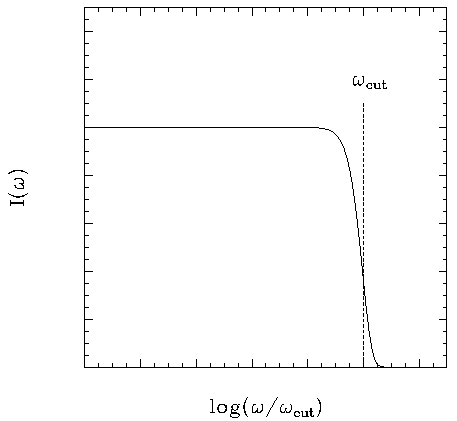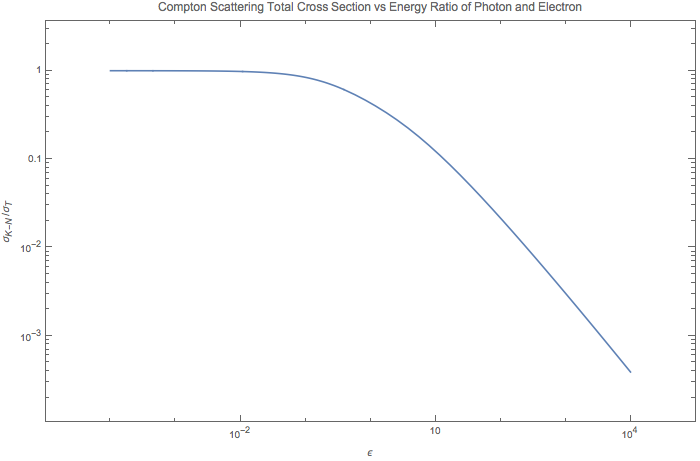# Astrophysics Basics¶

## Numbers¶

One of the most useful concepts in astrophysics is that things should be scaled using a scale that we can understand.

### Physical Facts¶

Ionization energy for atoms

1. H: 13.6eV
2. He: 24.7eV
3. $$He^{++}$$: 54.4eV

### Solar System¶

#### Sun¶

Some numbers:

1. Mass of Sun: $$M_\odot = 1.99 \times 10^{30}\mathrm{kg}$$;
2. Radius of Sun: $$6.9 \times 10^{5}\mathrm{km}$$;
3. Average Density: $$1.4\mathrm{g/cm^3}$$;
4. Surface Gravity: $$27 g_E$$;
5. Core Density: $$150\mathrm{g/cm^3}$$;
6. Core Temperature: $$1.5\times 10^7\mathrm{K}$$ which corresponds to energy of $$1\mathrm{keV}$$.

The sun is composed of

1. X = H mass fraction of $$0.70$$; 1. Y = He mass fraction of $$0.26$$; 1. X = Li mass fraction of $$0.04$$.

## Orbital Dynamics¶

Kepler’s law can be easily derived from Newton’s dynamics.

The first law is about the

## Wave Bands¶

A table of wavebands in astronomy is something like this.

## Doppler Shift¶

Refer to doppler-shift.

## Photon Production¶

There are two important processes that produces photons in astrophysical environment, namely thermal Bremsstrahlung and synchrotron radiation.Fig. 29 This is the spectrum of frequency-dependent emissivity of the process which happens when a flux of non-relativistic regime with thermal distributon of temperature T is shot into a plasma of ions or protons.

## Compton Scattering¶

The quantities we are interested in are cross section and radiated power, both of which are Lorentz invariant.

### Thomson Scattering¶

Total cross section of Thomson scattering can be obtained using only classical electrodynamics. The physics behind it is that the electric field excerts force on an electron then the electron emits photons to all possible direction as it oscillates.

The incoming power per unit area is

$P_{inc}= c u_{rad},$

as $$u_{rad} = \frac{E^2}{4\pi}$$ is the energy density of EM field.

The outgoing or scattered wave power (total) is

$P_{scatt} = \frac{2}{3}\frac{e^4}{m_e^2 c^3} E^2.$

The total cross section is ratio of the two quantity, i.e.,

$\sigma_T \equiv \frac{P_{scatt}}{P_{inc}} =\frac{8\pi e^4}{3 m_e ^2 c^4} = \frac{8\pi (\alpha \hbar c)^2}{3 m_e ^2 c^4},$

in which the fine structure constant is defined as $$\alpha = \frac{e^2}{\hbar c}$$.

### Compton Scattering¶

The full quantum electrodynamics result is called Klein-Nishima formula, which describes the total cross section of coliding photon and electron,

$\sigma_{K-N} = \frac{\pi e^4}{m_e^2 c^4} \frac{1}{\epsilon} \left[ \left(1 - \frac{2(\epsilon+1)}{\epsilon^2}\right) \ln (2\epsilon + 1) + \frac{1}{2} + \frac{4}{\epsilon} - \frac{1}{2(2\epsilon + 1)^2} \right],$

where $$\epsilon = \frac{E}{m_e c^2}$$.

In the limit that energy of electron is much larger than photons, we have $$\epsilon$$ is much smaller than 1, we would come back to the Thomson limit, which is true for our equation,

$\begin{split}\sigma_{K-N} &= \sigma_T \frac{3 \left(\left(1-\frac{2 (x+1)}{x^2}\right) \log (2 x+1)+\frac{4}{x}-\frac{1}{2 (2 x+1)^2}+\frac{1}{2}\right)}{8 x} \\ & = \sigma_T (1 - 2\epsilon + O(x^2)).\end{split}$

To have more understanding on this formula, I ploted $$\sigma_{K-N}$$ in terms of $$\sigma_T$$ as the energy scale $$\epsilon$$ changes.## Asteroseismology¶

The stars do shake, from inside out.

Long period variable such as Cepheids pulsate in the luminosity. This is because of the radial oscillation mode with a approximate period of

$P_{dynamical} \approx \left( \frac{R^3}{GM} \right)^{1/2} \approx (G\bar \rho)^{-1/2},$

in which we have the radius of the star as $$R$$, mass of the star as $$M$$ and mean density $$\bar \rho$$. The good thing of this oscillation immediately shows us the mean density of the star, even without any furthure inspectation.

There are double mode Cepheids, whose modes provides information about mass and radius.

Our sun, up to now we have identified thousands of individual modes. And more modes as many as $$10^{6}$$ modes can be determined accurately.[helioosc]_

### Papers, Researches and More¶

1. Double mode Cepheids, J. Otzen Petersen, 1973, 1974, 1978.
2. An introduction of seismology applied to stars. http://ap.smu.ca/~guenther/seismology/seismology.html